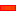﻿ Motion Law: For Quick-Return: 2': Zero Jerk @ Start/End'

# Throw - Quick-Return: 2: Zero Jerk @ Start/End/Mid-Point Motion-Law

### Throw: Quick-Return with Zero Jerk

The 'Quick-Return-2' motion-law is Not available in the Motion-Law Selector.

The 'Quick-Return-2' motion-law is a Rise & Return motion-law that is continuous in Velocity, Acceleration and Jerk.

Construct the 'Quick-Return 2' motion with two Flexible Polynomial Segments.

The first segment is the 'Rise', and the second the 'Return'. Each segment is a 'mirror' of each other.

Usually, the segments have an equal duration, but this is not necessary.

Continuity at the Mid-Point Blend-Point

The Position is maximum [P=1], and the Velocity is zero at the mid-point.

The Rise segment [below, in Blue] ends with a finite, negative acceleration.

The Return segment [below, in Red] starts with the same finite, negative acceleration.

There is acceleration continuity when the two segments follow each-other.

The Jerk is continuous and zero at the mid-point.

The Jerk is also zero at its start an end.

The position graph makes a quick-return at the mid-point because there is a large negative, but continuous, acceleration at the mid-point.

 • Quick Return: Finite Jerk @ Start/End/Mid-Point
 • Rapid Return: Finite Jerk @ Start/End/Mid-Point
 • Rapid Return: Smooth-Start/End

* An acceleration discontinuity would give a very poor dynamic response if applied to any mechanical system.

####Two Segment 'Quick-Return Motion 2'Quick-Return Motion 2' is designed with two Flexible-Polynomial Segments - usually of equal duration, but not necessarily.

Two Segments with Acceleration Continuity

Start: Rise Segment 1 @ Start

 • Position = 0
 • Zero Velocity
 • Zero Acceleration
 • Zero Jerk

Mid-Point: Segment 1 @ End = Segment 2 @ Start

 • Position = 1
 • Zero Velocity
 • Match, but unspecified Acceleration* [Actual = –40mm/s2]
 • Zero Jerk

End: Segment 2 @ End

 • Position 2 = 0
 • Zero Velocity
 • Zero Acceleration
 • Zero Jerk

* It is possible to reduce this slightly - to approximately 28, before it begins to make the motion-law less smooth.

####One Segment 'Rise Segment'Flexible Polynomial Parameters Settings

Start:

 • Position = 0
 • Zero Velocity
 • Zero Acceleration
 • Zero Jerk [Actual Jerk = 40mm/s3

End

 • Position = 1mm.
 • Zero Velocity
 • Unspecified* Acceleration [Actual Value = –10mm/s2]
 • Zero Jerk

### Motion Coefficients

Cv = 1.975

Ca = 5.120

Cd = –10 [Deceleration]

Cj max = 19.491

Cj min  = –39.402

Cj @ Crossover  = –35.556

Note: The Segment is two times longer than the Two Segment Motion above. The maximum motion values are: 1/2 of the Velocity,, 1/4 of the Acceleration, , 1/8 of the Jerk.

Tutorial and Reference Help Files for MechDesigner and MotionDesigner 13.2 + © Machine, Mechanism, Motion and Cam Design Software by PSMotion Ltd